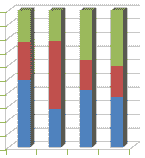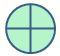Tutorial

# Rounding Number to 2 Decimal Places

In C#, you can round a number to two decimal places using various methods and techniques. One of the common ways to achieve this is by using the Math.Round() method. Here's how you can do it with examples:

## Using Math.Round() method

The Math.Round() method is used to round a decimal number to the nearest integer or specified number of decimal places. To round a number to two decimal places, you can use the overload of Math.Round() that accepts a second argument specifying the number of decimal places.

double number = 3.14159; // Round to two decimal places using Math.Round() double roundedNumber = Math.Round(number, 2); // Result: 3.14In this example, the variable number contains the value 3.14159. By using Math.Round(number, 2), the number is rounded to two decimal places, resulting in 3.14.

## Using String Formatting

Another approach to round a number to two decimal places is by using string formatting:

double number = 5.6789; // Round to two decimal places using string formatting string formattedNumber = string.Format("{0:0.00}", number); // Result: "5.68"

In this example, the string.Format() method is utilized with the format specifier "{0:0.00}" to round the number to two decimal places. The formatted result is "5.68".

## Using ToString() method with format

Alternatively, you can achieve the same result using the ToString() method with format specifier:

double number = 7.12345; // Round to two decimal places using ToString() method with format string formattedNumber = number.ToString("0.00"); // Result: "7.12"

In this example, the ToString() method with the format "0.00" is employed to round the number to two decimal places, yielding "7.12".

### ConclusionChoose the method that best suits your coding style and requirements. Keep in mind that rounding numbers can lead to potential inaccuracies due to the nature of floating-point representations. If precise decimal rounding is critical, consider using the decimal data type instead of double.